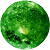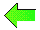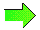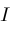ISSAC 94 - Symbolic-Numeric Nonlinear Equation SolvingContents News View

 AbstractA numerical equation-solving algorithm employing differentiation and interval arithmetic is presented which finds all solutions ofon an intervalwhenis holomorphic and has simple zeros.  A two dimensional generalization of this algorithm is discussed.  Finally, aspects of a broader symbolic-numeric algorithm which uses the first algorithm as a foundation are considered.